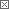Roger's Equations Blog### Roger's Equations

This blog is all about science and technology (with occasional math thrown in for fun). The goal of this blog is to try and pass on the sense of excitement and wonder I feel when I read about these topics. I hope you enjoy the posts.

 Previous in Blog: The Lagrangian - A Qualitative Analysis Next in Blog: The Hamiltonian - Quantum Mechanics# The Hamiltonian - A Qualitative Analysis

Posted March 22, 2007 2:34 PM by Bayes

In my last entry, we examined the Lagrangian,
L = T - V
where T is the kinetic energy and V is the potential energy of a closed system.
The Lagrangian was used by Joseph Louis Lagrange as part of an reformulation of classical mechanics.
The Lagrangian is found in the differential equation:where xi is just a generalized coordinate (could be x, y, z, r, θ, φ, etc.) andi is shorthand for dxi/dt which is velocity (v). The solution of which is the equation(s) of motion for the closed system.

The reason that the energies of a system predict the equations of motion introduces us to the Principle of Least action which essentially says that nature wants to convert potential energy to kinetic energy or vice-versa by the most efficient means possible with respect to time. The motion of a system is simply the physical manifestation of this principle of least action.
The lagrangian is a function expressed in terms of position (x) and velocity () as well as time. This is because kinetic energy is velocity dependent, e.g. T = 1/2mv2 , and potential energy is position dependent, e.g V=1/2kx2 and the Lagrangian is the difference of the kinetic energy and potential energy.

The Hamiltonian
The Hamiltonian is a reformulation of the classical mechnaics by William Rowan Hamilton, just like the Lagrangian, where instead of velocity, momentum is used and instead of the difference of the kinetic and potential energies, the sum is used. It is derived from the Lagrangian,where the qi is just a different notation for generalized coordinates (means the same exact thing as xi ) and pi is the generalized momentum (could be Px, Py, Pz, Pr, etc. where Px= m= mvx , Py=mvy etc.).

and
Px = dL/dThe equation above for the Hamiltonian is always a lot to take in a first glance, so I think it's easier to understand with example.

Let's take the system from last entry,
Example
Consider an object at height x above the Earth initially at rest. The Potential Energy (V) of that object would be V=mgx. The Kinetic Energy (T) of a mass once it starts to fall will be 1/2mv2. So,

L = T - V = 1/2mv2 - mgx
so from the equation:

p = dL/dwe get;

p = mv or if we reorder, v=p/m
which we use in the equation;

H(p,x,t) = vp - L(v,x,t)
to get

H(p,x,t) = (p/m)p - 1/2m(p/m)2 + mgx
H(p,x,t) = P2/m - P2 /2m + mgx
H(p,x,t) = P2/2m + mgx
but p=mv, so the Hamiltonian, besides being a reformulation of the Lagrangian in terms of momentum, also is more importantly:

H(p,x,t) = m2v2/2m + mgx
H(v,x,t) = 1/2 mv2 + mgx
H(T,V) = T + V
The sum of the kinetic energy and the potential energy, the total energy of the system.
So what is the Hamiltonian?
The Hamiltonian is the sum of the Kinetic and Potential Energy of a closed system expressed in terms of momentum, position, and time.

in contrast to the Lagrangian;

The Lagrangian is the difference of the Kinetic and Potential Energy of a closed system expressed in terms of velocity, position, and time.
Once you have the Hamiltonian, you can get Hamilton's equations of motion, namelyfrom the example above, our Hamiltonian is
H(p,x,t) = P2/2m + mgx

so

v = P/m

and
-dp/dt = mg
p=-mgt
which if we substitute into the first equation of motion;

v=P/m

gives

v=-gt
which is exactly what we got from the Lagrangian. The Hamiltonian is just a different way of getting the equations of motion of a system.
Conclusions
So the Lagrangian and Hamiltonian are two ways of doing the same thing, namely they are functions that track the potential and kinetic energies of a closed system. The equations of motion come from applying the principle of least action to these functions.
Next time I will explain the Hamiltonian as it appears in quantum mechanics, which is an extension of the classical Hamiltonian to a world where one cannot know both the position and momentum of a system at the same time.
Special thanks to the following websites:
http://www.mathpages.com/home/kmath523/kmath523.htm
http://en.wikipedia.org/wiki/Hamilton%27s_equations
http://fqxi.org/community/forum/topic/1388

Interested in this topic? By joining CR4 you can "subscribe" to